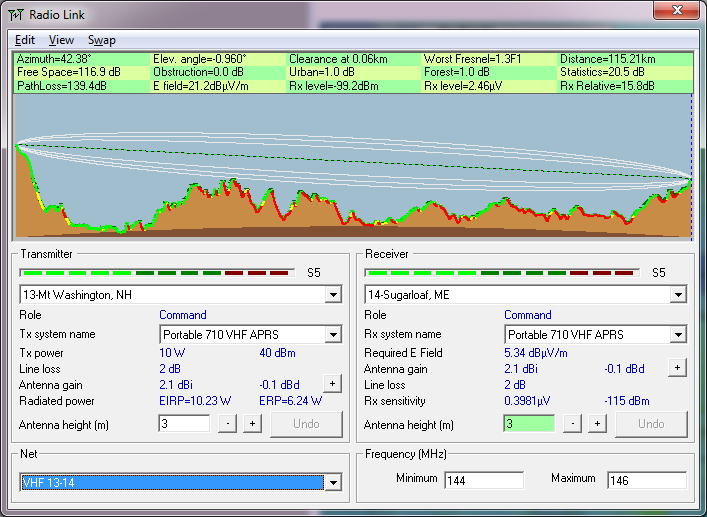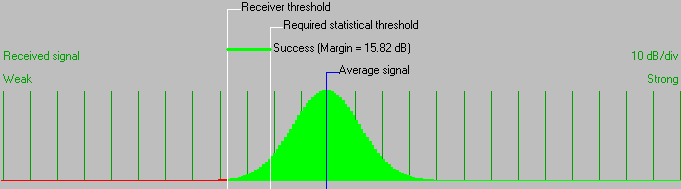• Distance between 13-Mt Washington, NH and 14-Sugarloaf, ME is 115.2 km (71.6 miles)
• True North Azimuth = 42.38°, Magnetic North Azimuth = 57.66°, Elevation angle = -0.9602°
• Terrain elevation variation is 1660.3 m
• Propagation mode is line-of-sight, minimum clearance 1.3F1 at 0.1km
• Average frequency is 145.000 MHz
• Free Space = 116.9 dB, Obstruction = 0.0 dB, Urban = 1.0 dB, Forest = 1.0 dB, Statistics = 20.5 dB
• Total propagation loss is 139.4 dB (interference mode (optimistic))
• System gain from 13-Mt Washington, NH to 14-Sugarloaf, ME is 155.2 dB
• System gain from 14-Sugarloaf, ME to 13-Mt Washington, NH is 155.2 dB
• Worst reception is 15.8 dB over the required signal to meet 95.000% of situations